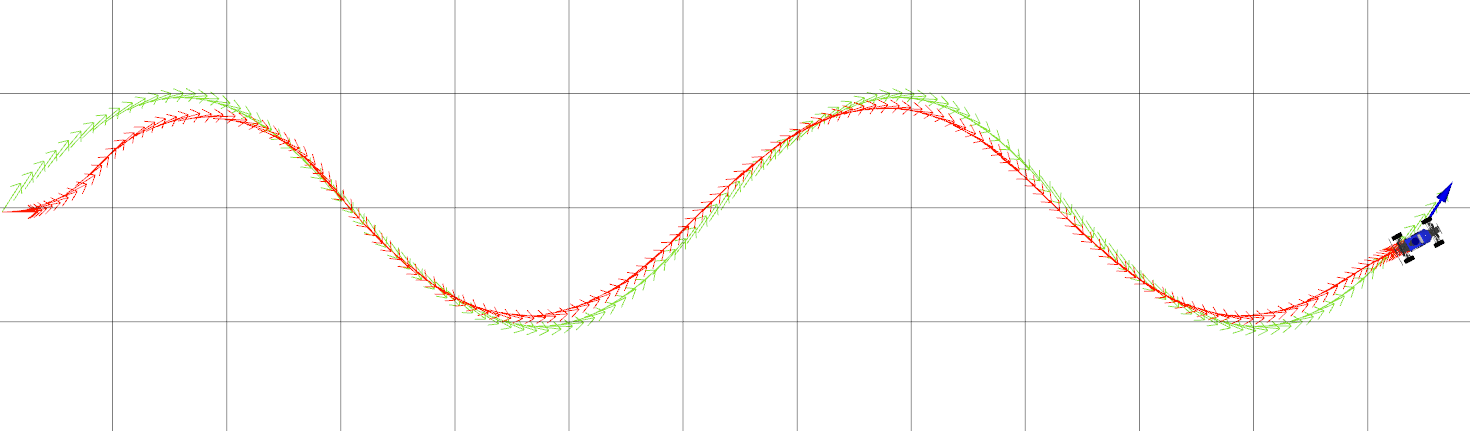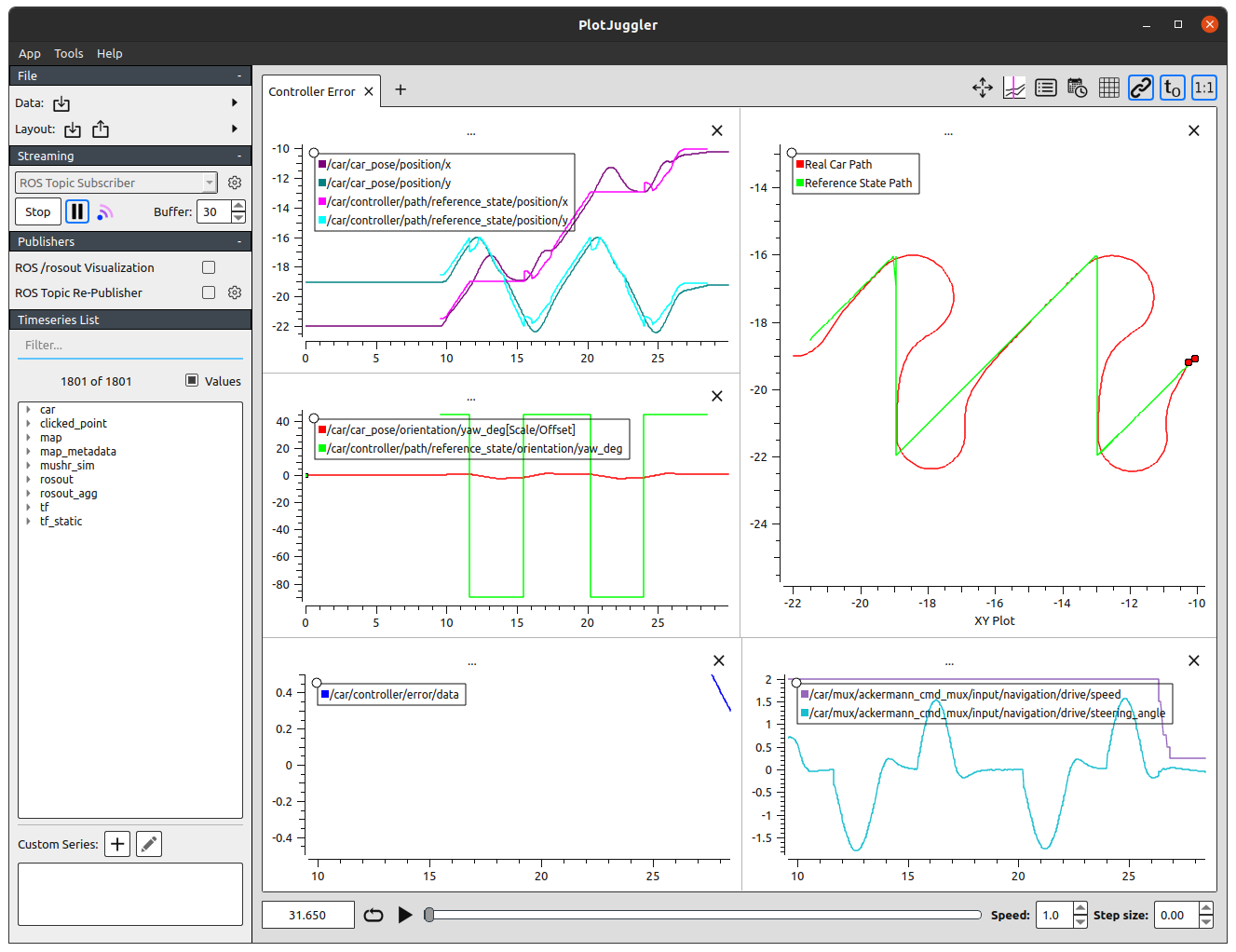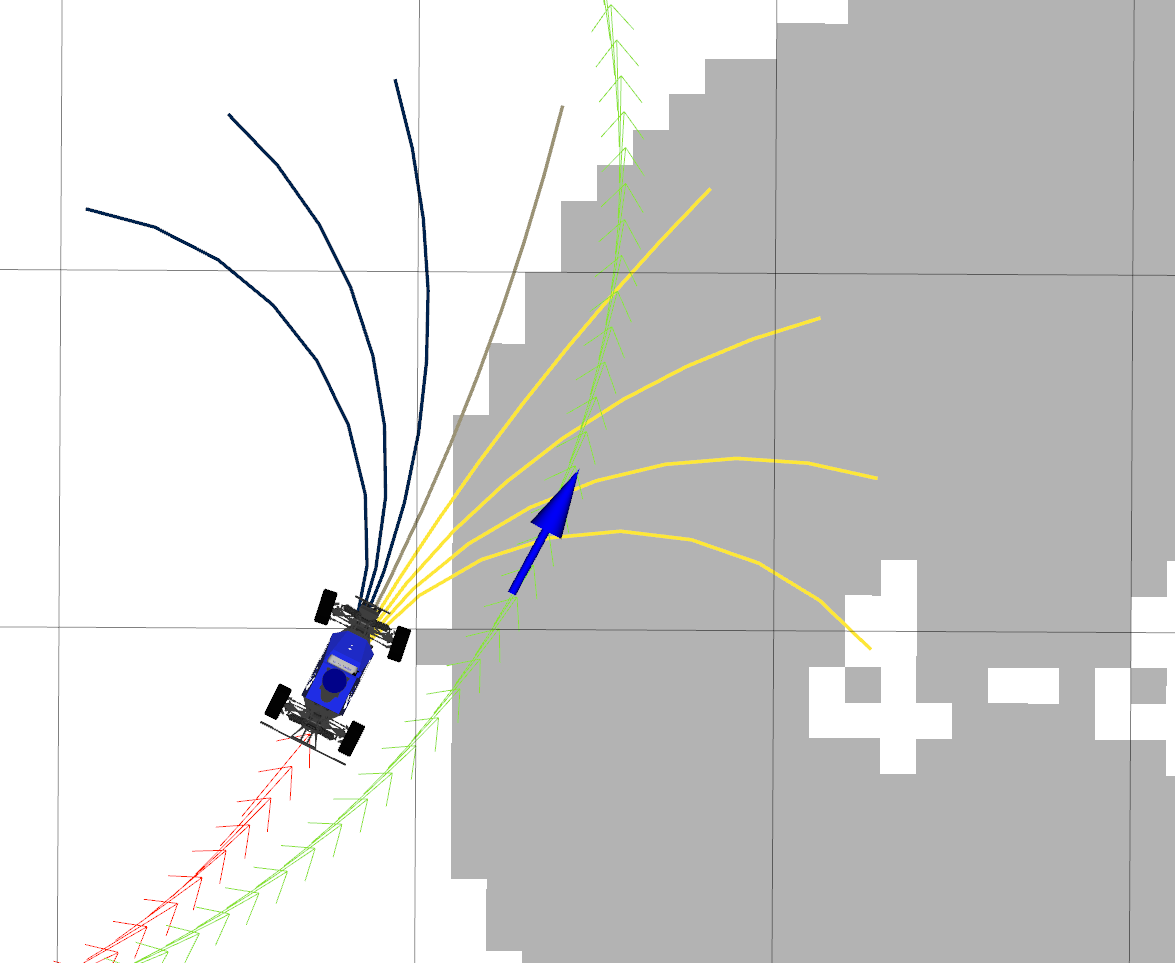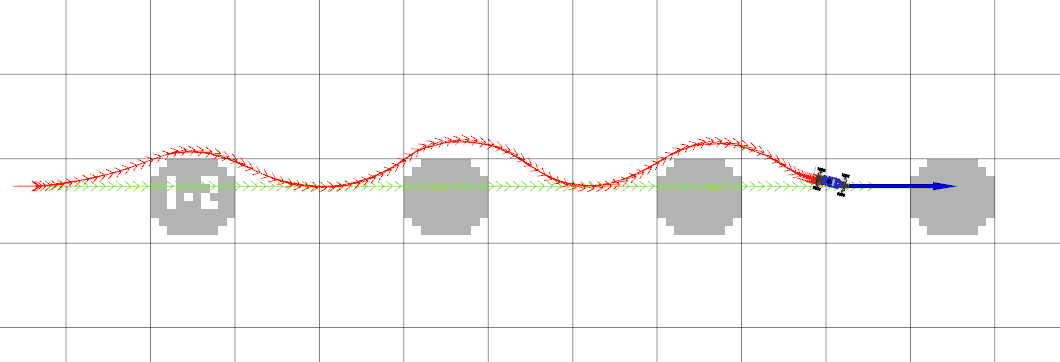# Project 3: Control## Overview

In this project, you will implement several fundamental path tracking control algorithms: PID, Pure Pursuit, and MPC.1 To accurately track the desired paths, you will tune the parameters that govern their behavior. As a result, you will also become familiar with the strengths and weaknesses of the various feedback control strategies discussed in this course.

This project is designed for a group of 2-3 students, but may also be completed individually. Each group should write their own writeup and code. If you need to change your group, please let the course staff know on Ed.

The relevant lectures are:

• Lecture 6: Introduction to Feedback Control
• Lecture 7: PID Control
• Lecture 8: Pure Pursuit and Model-Predictive Control

## Getting Started

We’ve updated the MuSHR dependencies since Project 2, so you’ll need to pull and rebuild both of your workspaces. (This is the same procedure that you did for Project 2.) The order of the steps is important, so please follow them precisely and restart if you accidentally skip a step.

From a fresh terminal:

$source /opt/ros/noetic/setup.bash$ # Update and fresh-build dependencies
$cd ~/dependencies_ws/src$ catkin clean
$cd cse478_dependencies$ git pull
$git submodule update --init --recursive$ # Install new binary dependencies (there will be one warning you can ignore)
$rosdep install --from-paths . --ignore-src -y -r$ cd ..
$catkin build$ source ~/dependencies_ws/devel/setup.bash

The next steps depend on whether you’re using the same individual repository as Project 2, or whether you have a new repository. Please follow the corresponding instructions.

Same Repository as Project 2

If you’re using the same repository, you’ll need to pull down the latest version of the starter repository to get the Project 3 starter code. You’ll do that by adding our starter repository as a new remote repository, named upstream. Then, you’ll pull the files from this upstream remote repository into your local repository.

$cd ~/mushr_ws/src/$ catkin clean
$cd mushr478$ git remote add upstream https://gitlab.cs.washington.edu/cse478/21sp/mushr478.git
$git pull upstream main$ # Install new binary dependencies
$rosdep install --from-paths . --ignore-src -y -r$ cd ..
$catkin build$ source ~/mushr_ws/devel/setup.bash
Different Repository than Project 2

If you’re using a new repository, it’s already been populated with our Project 3 starter code. But first, we need to deal with your old repository.

Rename your old repository, so you can follow the paths as written in this project with your new repository. Then, you’ll have to tell Catkin to ignore your old repository (or there will be name conflicts between the packages in your old and new repository). You’ll do that by adding an CATKIN_IGNORE file to your old repository.

Then, you’ll clone your fresh new repository.

$cd ~/mushr_ws/src/$ catkin clean
$# Rename your old repository and tell Catkin to ignore it$ mv mushr478 mushr478-netid
$touch mushr478-netid/CATKIN_IGNORE$ # Clone your new repository
$git clone git@gitlab.cs.washington.edu:cse478/21sp/your_new_repo_name.git mushr478$ # Install new binary dependencies
$rosdep install --from-paths . --ignore-src -y -r$ cd ..
$catkin build$ source ~/mushr_ws/devel/setup.bash

If the builds succeed and you can run roscd control, you’re ready to start! Please post on Ed if you have issues at this point.

## Code Overview

We decompose the problem of computing the robot’s actions into two components: motion planning and control. A motion planning algorithm generates an ideal reference path, while a feedback controller uses the robot’s current state (e.g. from the localization package) to follow this reference path. In this project, we will use a series of pre-programmed paths; you will implement motion planning algorithms in the next project.

We’ve provided a BaseController class in src/control/controller.py. The controllers you will implement (PIDController, PurePursuitController, ModelPredictiveController) extend this base class.

The ControlROS class in src/control/control_ros.py provides a ROS interface to the controller classes. ControlROS provides a ROS service defined by FollowPath.srv. This service accepts a request (consisting of a nav_msgs/Path and desired speed) and asks the controller to track the geometric path at that speed. While the controller is tracking the path, ControlROS also publishes to several visualization topics.

The control loop is implemented in BaseController. BaseController.get_reference_index selects a reference state from the path. Each subclass implements controller-specific logic in the get_error and get_control methods. In order to operate at the desired control rate2, your implementations of all three methods must be efficient. Try to vectorize where you can!

The reference paths being tracked by your controllers have reference velocities, and are represented as NumPy arrays of shape (L, 4). Paths with velocity information are named path_xytv, while paths without velocity information are named path_xyt.3 Similarly, a reference state with velocity information is named reference_xytv.

The control vectors returned by get_control contain both velocity and steering angle. In this project, the velocity should be copied directly from the reference velocity. The commanded steering angle will come from your PID/Pure Pursuit/MPC logic.

Implementing MPC will require more time than the other two controllers. Please plan accordingly.

## Q1. Reference State Selection and Error Computation

Q1.1 Implement the BaseController.get_reference_index method to select a reference state from the path that is about distance_lookahead from the current robot state (src/control/controller.py). This will be used as the target state for your controllers. To avoid selecting a reference state that would take the robot backwards, you should first compute the closest waypoint on the path and only consider states on the path that come after this closest waypoint.

To compute a reference state that is some lookahead distance away, we recommend first finding the state on the path that is closest to the current vehicle state. Then, step along the path’s waypoints and select the index of the first state that is greater than the lookahead distance from the current state. (You can also look back one index to see which state is closer to the desired lookahead distance.) If no such state exists, you can simply return the final state.

You can verify your implementation on the provided test suite by running python3 test/controller.py. Your code should pass all test cases starting with test_get_reference_index.

Q1.2: Implement the compute_position_in_frame function in src/control/controller.py. You may find the “Position in Frame B” slide from Lecture 7 (Slide 14) or the following derivation useful. This function will be used by your PID and Pure Pursuit controllers: the PID error is the current robot position $$(x, y)$$ expressed in the coordinate frame defined by $$(x_\text{ref}, y_\text{ref}, \theta_\text{ref})$$, while the Pure Pursuit error is the position $$(x_\text{ref}, y_\text{ref})$$ expressed in the coordinate frame defined by $$(x, y, \theta)$$.

Computing Position in a New Coordinate Frame

Let $$\mathbf{p} = (x, y)$$ be the position that we want to be expressed in a new coordinate frame $$F$$ defined by the state $$(x_F, y_F, \theta_F)$$. Then, $$\mathbf{p}$$ expressed in frame $$F$$ is $${^F}\mathbf{p}$$.

\begin{align} {^F}\mathbf{p} = R(-\theta_F) \left( \begin{bmatrix} x \\ y \end{bmatrix} - \begin{bmatrix} x_F \\ y_F \end{bmatrix} \right) = \begin{bmatrix} \cos \theta_F & \sin \theta_F \\ -\sin \theta_F & \cos \theta_F \end{bmatrix} \left( \begin{bmatrix} x \\ y \end{bmatrix} - \begin{bmatrix} x_F \\ y_F \end{bmatrix} \right) \end{align}

After completing Q1.1 and Q1.2, expect your code to pass all the test cases in test/controller.py.

## Q2. PID Controller

The proportional-integral-derivative (PID) controller is a feedback control mechanism that is simple to implement and widely used. It is often defined as $u(t) = -\left( K_p e(t) + K_i \int_0^t e(t') dt' + K_d \frac{d e(t)}{dt} \right)$ where $$K_p$$, $$K_i$$, and $$K_d$$, are all non-negative coefficients for the proportional, integral, and derivative terms of the error $$e(t)$$ at time $$t$$. In this project, we will actually implement a PD controller, which drops the integral term.

Q2.1: Implement the PD control law in PID.get_control for $$e(t) = e_\text{ct}$$. Note that the error argument is the result of get_error, a two-element NumPy array $$[e_\text{at}, e_\text{ct}]$$. The returned control should be a two-element NumPy array $$[v, \delta]$$ where $$v$$ is copied from the reference velocity and $$\delta$$ is the result of the PD control law. Hint: recall from lecture that the derivative of the cross-track error can written analytically as $$\frac{d e_\text{ct}}{dt} = v \sin (\theta - \theta_\text{ref})$$.

You can verify your implementation on the provided test suite by running python3 test/pid.py.

### Exploring PID Parameters

The PID controller is controlled by the gains $$K_p, K_i, K_d$$. We’ve provided some initial values in config/parameters.yaml; note that $$K_i = 0$$ since our implementation is a PD controller. As with the previous project, it’ll be up to you to tune the gains $$K_p, K_d$$ to achieve good path tracking performance. This Wikipedia article describes a principled approach to finding robust PID gains.4 You may also find this blog post useful; the notation is somewhat different, but it describes a number of tracking controllers and you can use the sliders to build your intuition for how each gain affects the controller.

Tune your gains $$K_p, K_d$$ to achieve good tracking performance. In your writeup, briefly explain your tuning process, justify your final gains, and include the plots generated by PlotJuggler for three reference paths: circle, left-turn, and wave. Suggested names for these plots: pid_circle.png, pid_left.png, pid_wave.png. (Instructions for sending reference paths are in the next section.)

After tuning your gains, evaluate your implementation on the different paths with the following test. Your implementation is expected to track each path within a specified error threshold.

$rostest control controller_performance.test type:=pid ## Controller Visualization Tools To evaluate your controller with reference paths, we’ll launch our environment across a few terminals. Open one terminal and run: $ roslaunch cse478 teleop.launch teleop:=false

In another, launch a controller (with your choice of pid, pp, or mpc):

$roslaunch control controller.launch type:=pid Now, to see the controller node’s visualization data: $ rosrun rviz rviz -d $(rospack find control)/config/control.rviz Finally, send a reference path to the controller. The available path names are: line, circle, left-turn, right-turn, wave, and saw. (saw is a challenging path, so don’t worry if your controllers can’t track it very well.) $ rosrun control path_sender line

In the RViz window, you should see a visualization of the reference path you’ve selected, as well as how the MuSHR car moves according to the PD controller. Here is a snapshot of the staff solution PD controller tracking the default wave path. To test the robustness of your controller, you can also vary the parameters of the paths and the initial state of the car. Learn more about the path parameterizations by running path_sender with the -h flag.Figure 1: The MuSHR car tracking the default wave path with the staff PD controller.

While you’re implementing your controller, it can be helpful to see a plot of its output over time. We have including a configuration for PlotJuggler, a time series visualization tool, that lets you see the error and controls calculated by the controller in real time. Run the following command to start.

$roslaunch control plotjuggler.launch When PlotJuggler starts up, you’ll have to answer two questions fairly quickly so that it starts while the path is still being tracked. Click yes when it asks “Start the previously used streaming plugin? ROS Topic Subscriber” and select all the ROS topics that it asks about (Ctrl-A). If you see a warning about “Can’t find one or more curves.”, just close PlotJuggler and start again. Once PlotJuggler has been completely initialized, you can issue more paths and see the plots in real-time! When a path is complete, press the pause button on the left. You might see something similar to this.Figure 3: The MuSHR car tracking the default saw path, as seen through PlotJuggler. If PlotJuggler isn’t already installed on your virtual machine, you can also run this command. $ sudo apt install ros-noetic-plotjuggler-ros
Installing PlotJuggler from source

PlotJuggler may not work well for certain versions of OpenGL. You can install an updated version of the tool, which allows you to disable OpenGL.

First, clone and compile the PlotJuggler repositories.

$cd ~/mushr_ws/src$ git clone https://github.com/PlotJuggler/plotjuggler_msgs.git
$git clone https://github.com/facontidavide/PlotJuggler.git$ git clone https://github.com/PlotJuggler/plotjuggler-ros-plugins.git
$rosdep install --from-paths . --ignore-src -y -r$ cd ..
$catkin build$ source ~/mushr_ws/devel/setup.bash

After PlotJuggler has started up, open the preferences menu with “App > Preferences” and disable OpenGL.

## Q3. Pure Pursuit Controller

Pure Pursuit is a classic feedback control mechanism for autonomous vehicles that calculates the circular arc between the robot’s current state and the reference state. In general, the lookahead distance used to select the reference state may change as the robot drives to reflect the curvature of the path, although your implementation will assume a fixed lookahead distance.

Q3.1: Implement the Pure Pursuit control law in PurePursuit.get_control. As before, the error argument is the result of get_error.

You can verify your implementation on the provided test suite by running python3 test/purepursuit.py.

### Exploring the Pure Pursuit Lookahead

The Pure Pursuit controller only has one parameter to tune: the distance_lookahead to select the reference state. As before, we’ve provided an initial value in config/parameters.yaml that needs to be tuned to achieve good path tracking performance.

To visualize the controller, follow the same steps from above but with the Pure Pursuit controller instead. As before, you are encouraged to vary the parameters of the paths and initial state of the car to test the robustness of your controller.

$roslaunch control controller.launch type:=pp Follow the same steps from above to evaluate the tracking performance of your tuned Pure Pursuit controller. $ rostest control controller_performance.test type:=pp

Tune the lookahead distance to achieve good tracking performance with Pure Pursuit. In your writeup, justify your final lookahead distance by including the controller plots for the wave reference path (pp_wave.png). Additionally, include plots for the wave path where the lookahead distance is too small/large and explain the resulting Pure Pursuit behavior (pp_small.png, pp_large.png).

Evaluate the tracking performance on the circle path with radius parameters 5 and 0.5: rosrun control path_sender circle --radius <R>. How does varying the radius affect the robustness of your controller? Why?

To learn more about Pure Pursuit, you may also find the following supplementary readings interesting. (Coulter, 1992) is the original Pure Pursuit paper, which describes the algorithm and its history. (Paden et al., 2016) is a recent survey paper that describes a number of popular control techniques for vehicles, including Pure Pursuit.

## Q4. Model-Predictive Controller (MPC)

Although the PID and Pure Pursuit controllers you’ve implemented can be adequate in some driving conditions, additional factors may affect path tracking in practice. For example, nearby obstacles may require segments of the path to be tracked more closely, but are not incorporated into these simple controllers. More generally, these controllers are myopic: they reason solely about the current action and do not predict how that might impact future actions.

MPC proceeds in two phases. First, it uses a model to solve an $$T$$-horizon optimization problem. This problem is defined by a cost function $$J$$ that penalizes states and actions (e.g., states that are in collision); the solution is a sequence of $$T-1$$ actions that minimizes cost. Next, the system will execute the first action and update the current estimated state. The process repeats from this new estimated state.

In your implementation, we will separate the optimization into four steps.

1. Sample $$K$$ sequences of $$T-1$$ controls (sample_controls).
2. Use the kinematic car model from Project 2 to rollout the resulting $$T$$-length sequence of states, including the current estimated state (get_rollout).
3. Compute the cost of each rollout from the $$T$$ resulting states (compute_rollout_cost).
4. Of the $$K$$ rollouts, select the rollout with the lowest cost and return the first action from this sequence (get_control).

Q4.1: Implement MPC.sample_controls. This function should return a three-dimensional NumPy array with shape (K, T-1, 2), representing $$K$$ sequences of $$T-1$$ controls (velocity and steering angle). In your implementation, each of the sequences corresponds to a particular steering angle, applied $$T-1$$ times. The steering angles in the $$K$$ sequences should evenly span the range of the steering angle, defined by self.min_delta and self.max_delta. (Note that the velocity returned by this method is a placeholder, which will be replaced by the reference velocity in the next question. This is computed once per path tracking request for efficiency.)

After completing Q4.1, expect your code to pass the test_sample_controls test case in test/mpc.py.

Q4.2: Implement MPC.get_rollout. This function should return a three-dimensional NumPy array with shape (K, T, 3), representing $$K$$ sequences of $$T$$ resulting states $$(x, y, \theta)$$. The first pose of each rollout should always be the current pose. To compute the change in state for each step of the rollout, call self.motion_model.compute_changes (which you implemented in Project 2). Remember to vectorize your implementation as much as you can!

After completing Q4.2, expect your code to pass the test_get_rollout test case in test/mpc.py.

Q4.3: We’ve implemented MPC.compute_rollout_cost to call two components of the cost function: MPC.compute_distance_cost and MPC.compute_collision_cost. This cost function penalizes the rollout based on the distance between the final state and the reference state, as well as how many of the states in the rollout would result in collisions. The distance should be weighted by self.error_w and the collision cost should be weighted by self.collision_w. Implement both of these methods. (You should not change compute_rollout_cost.)

After completing Q4.3, expect your code to pass the cost-related test cases in test/mpc.py.

Tuning the parameters of a cost function requires principled design, iteration, and testing. We’ve asked you to implement a specific cost function with two terms and pre-specified weights, but these are all parameters that would be tuned in practice.Figure 4: MPC rollouts, colored by cost. Yellow rollouts have high cost due to collisions and blue have low cost.

Q4.4: Implement MPC.get_control to solve the $$T$$-horizon optimization problem. We’ve provided the logic for setting self.sampled_controls to match the reference velocity, so it’s ready for computing the rollout. Call the methods you’ve already implemented to fill in values for rollouts and costs, select the rollout with the lowest cost, and return the first action from this sequence.

### Exploring MPC Parameters

MPC is controlled by the parameters K and T in config/parameters.yaml, which you should tune to achieve good path tracking performance. In particular, MPC should now allow you to track paths around obstacles that aren’t accounted for by the planner (Figure 5). (The reference paths from path_sender don’t consider obstacles at all.)

We’ve also provided a fun new map under cse478/maps for you to play around in: slalom_world. MPC should be able to navigate around these obstacles! Start the simulator with this new map.

$roslaunch cse478 teleop.launch teleop:=false map:='$(find cse478)/maps/slalom_world.yaml'

You can set different initial positions with the “Publish Point” RViz tool to try navigating around different slaloms. Then, launch MPC and issue a reference path. As before, you may want to evaluate your controller with different path parameters.Figure 5: MPC avoiding obstacles in the slalom_world map while tracking the line path.

Follow the same steps from above to evaluate the tracking performance of your tuned MPC implementation.

$rostest control controller_performance.test type:=mpc Tune the MPC parameters $$K$$ and $$T$$ to achieve good tracking performance. In your writeup, briefly explain your tuning process and justify your final parameters. Include the controller plots for the circle, wave, and saw reference paths in the default sandbox map (mpc_circle.png, mpc_wave.png, mpc_saw.png). What makes the saw path so difficult to track? Include the controller plots for two reference paths and slaloms of your choice in the slalom_world map. ## Deliverables Answer the following writeup questions in control/writeup/README.md. All figures should be saved in the control/writeup directory. For the controller plots, you may submit either an image of PlotJuggler or a screenshot of RViz after the path has completed. 1. What tradeoffs did varying the $$K_p$$ gain have on your PD controller’s performance? 2. Describe the tuning process for your PD controller. Justify your final gains $$K_p, K_d$$ and include the controller plots for three reference paths on the default sandbox map (pid_circle.png, pid_left.png, pid_wave.png). 3. Describe the lookahead distance tuning process for your Pure Pursuit controller. Justify your final lookahead distance by including the controller plot for the wave reference path on the default sandbox map (pp_wave.png). 4. Include controller plots on the wave path for cases where the lookahead distance is too small/large (pp_small.png, pp_large.png). Explain the resulting Pure Pursuit behavior. 5. How does varying the radius of the circle path affect the robustness of the Pure Pursuit controller? 6. Describe the tuning process for the MPC optimization parameters. Justify your final parameters $$K, T$$ by including the controller plots for the circle, wave, and saw reference paths on the default sandbox map. What makes the saw path so difficult to track? 7. Include controller plots for two reference paths and slaloms of your choice in the slalom_world map. 8. In this project, we asked you to implement a very specific MPC cost function that only includes distance and collision terms (and specific weights for the two terms). What other terms might you include, if you were to customize your cost function? (You don’t have to implement this, just describe some ideas.) 9. Of your three tuned controllers, in which settings is each controller most robust? Which controller worked best in high-speed regimes? You can set the desired speed by adding the --speed <SPEED> flag to path_sender. ## Submission As with the previous projects, you will use Git to submit your project (code, writeup and plots). From your mushr478 directory, add all of the writeup files and changed code files. Commit those changes, then create a Git tag called submit-proj3. Pushing the tag is how we’ll know when your project is ready for grading. $ cd ~/mushr_ws/src/mushr478/
$git diff # See all your changes$ git add .                            # Add all the changed files
$git commit -m 'Finished Project 3!' # Or write a more descriptive commit message$ git push                             # Push changes to your GitLab repository
$git tag submit-proj3 # Create a tag named submit-proj3$ git push --tags                      # Push the submit-proj3 tag to GitLab

## Optional: Running on the Car

Instructions coming soon.

1. The controllers you will implement have been used in the DARPA Grand Challenge and DARPA Urban Challenge among many other scenarios.↩︎

2. Our PID and Pure Pursuit implementations operate at ~50 Hz. Our tuned MPC operates at ~25 Hz, trading off a higher control frequency for a longer search horizon.↩︎

3. A path_xyt is a converted nav_msgs/Path message, represented as a NumPy array of shape (L, 3). ControlROS adds velocity information to create path_xytv before sending that to your controllers.↩︎

4. Note that this approach is designed to tune $$K_i$$ as well, so it may not perform quite as well when only given $$K_p$$ and $$K_d$$ to tune.↩︎